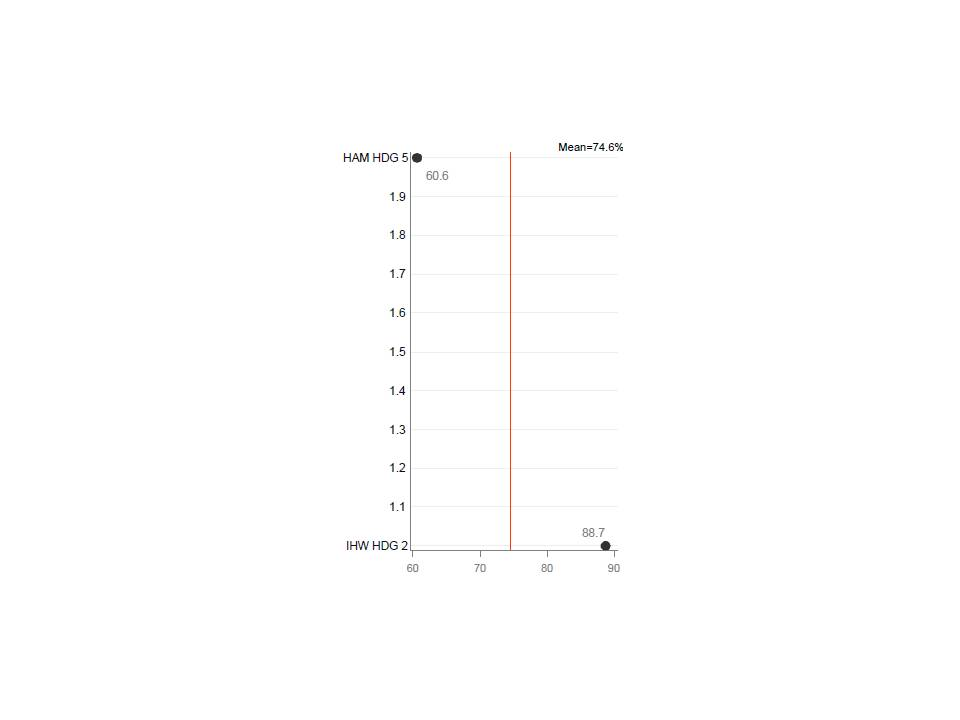## GPlot y axis labeling

I'm producing the graph below with this (pared down) code:

axis1 minor=none label=none;

axis2 minr-none major=none label=none;

proc gplot;

format n2 lbl.;

plot n2*col1/haxis=axis1

vaxis=axis2

href=74.6;

run;

When there are more than 2 values on the y axis, only the formatted values appear.  With 2 values (e.g. 1 and 2), the values in between also appear as shown.  How do I stop the 1.1 to 1.9 values from showing up on the y axis?

Thanks,

Bill1 ACCEPTED SOLUTION

Accepted Solutions

## Re: GPlot y axis labeling

proc gplot data=have;

format n2 lbl.;

plot n2*col1/haxis=60 to 90 by 10

vaxis=1 to 2 by 1
href=74.6;
run;

6 REPLIES 6

## Re: GPlot y axis labeling

Use a where statement.

proc gplot;

where n2=1 or n2=2;

format n2 lbl.;

plot n2*col1/haxis=axis1

vaxis=axis2

href=74.6;

run;

## Re: GPlot y axis labeling

proc gplot data=have;

format n2 lbl.;

plot n2*col1/haxis=60 to 90 by 10

vaxis=1 to 2 by 1
href=74.6;
run;DanH_sas
SAS Super FREQ

## Re: GPlot y axis labeling

You might want to try PROC SGPLOT to handle this situation. There is an option on SGPLOT's axis statements called INTEGER that should do exactly what you want. Your code above would look something like the following with SGPLOT:

proc sgplot data=sample;

format n2 lbl.;

yaxis display=(noticks nolabel) integer;

xaxis display=(nolabel);

scatter y=n2 x=col1;

refline 74.6 / axis=x;

run;

You need at least SAS 9.2 to run this.

Hope this helps!

Dan

## Re: GPlot y axis labeling

axis2 minr-none major=none label=none;

axis2 minor=none major=none label=none;

## Re: GPlot y axis labeling

Pronabesh's 2nd solution is correct.

Or, you might want to use a separate axis statement, to allow a little more flexibility, such as ...

axis1 order=(1 to 2 by 1);

axis2 order=(60 to 90 by 10);

proc gplot data=have;

format n2 lbl.;

plot n2*col1 / haxis=axis2 vaxis=axis1 href=74.6;
run;

Details:

The reason you're seeing the extra values along the vertical axis is that gplot just sees it as a numeric axis, and therefore picks a good numeric range to encompass the min-to-max numeric values, and adds some major tickmarks between the min & max for good measure (the default is to always have several tickmarks along the numeric axis). When you specify the axis order "by 1", then only the integer major tickmarks are used (no 1.1, 1.2, 1.3, etc).

## Re: GPlot y axis labeling

Thank you Pronabesh and Robert.  Your responses are indeed correct.  I was hoping for something a little more generic so that I could avoid some macro programming - this situation uses a by statement within gplot where the y axis levels vary in number (2 to N).  I'll have to write some code to create a &max in place of "2" in the example.

Discussion stats
• 6 replies
• 2269 views
• 6 likes
• 5 in conversation